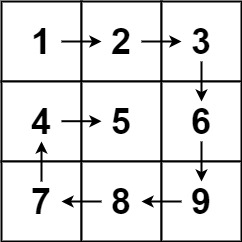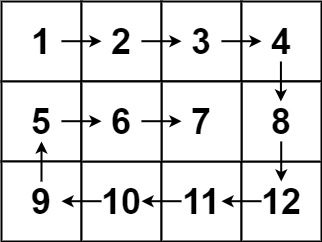# 54. Spiral Matrix LeetCode

## 54. Spiral Matrix

Given an `m x n` `matrix`, return all elements of the `matrix` in spiral order.

Example 1:```Input: matrix = [[1,2,3],[4,5,6],[7,8,9]]
Output: [1,2,3,6,9,8,7,4,5]
```

Example 2:```Input: matrix = [[1,2,3,4],[5,6,7,8],[9,10,11,12]]
Output: [1,2,3,4,8,12,11,10,9,5,6,7]
```

Constraints:

• `m == matrix.length`
• `n == matrix[i].length`
• `1 <= m, n <= 10`
• `-100 <= matrix[i][j] <= 100`

### The solution to the above question

class Solution {
public:
vector<intspiralOrder(vector<vector<int>>& v) {
int SR=0,ER=v.size()-1;
int SC=0EC=v.size()-1;
int count=0;
int a=(ER+1)*(EC+1);
cout<<a;
vector<int>ans;
while(SR<=ER && SC<=EC){
for(int i=SCi<=ECi++){
ans.push_back(v[SR][i]);
count++;
if(count==a){
return ans;
}
}
SR++;

for(int i=SR;i<=ERi++){
ans.push_back(v[i][EC]);
count++;
if(count==a){
return ans;
}
}
EC--;

for(int i=ECi>=SCi--){
ans.push_back(v[ER][i]);
count++;
if(count==a){
return ans;
}
}
ER--;

for(int i=ERi>=SRi--){
ans.push_back(v[i][SC]);
count++;
if(count==a){
return ans;
}
}
SC++;

}
return ans;
}
};